## Geometric Dimensioning and Tolerancing Quiz ASME Y14.5 – 2009

#### So you think you know Geometric Dimensioning and Tolerancing (GD&T)? Take our real-time GD&T quiz. You’ll receive your score immediately after completing the quiz.

Applied Geometrics (AGI) offers GD&T Training in several convenient formats to advance your GD&T proficiency, including live seminars, webinars, and convenient self-paced online training. Take our exam below to see how well you know GD&T and how prepared you are for the ASME GD&T exam:

This test is for the evaluation of knowledge and skills in Geometric Dimensioning & Tolerancing per the ASME Y14.5 -2009 standard.

1. Which statement best describes what the ASME-Y14.5 - 2009 covers?

2. Using GD&T:

3. How can properly implemented GD&T save money in the manufacturing process?

4. What is a Basic Dimension?

5. What is a Feature Control Frame?

### Select the description for each Geometric Characteristic Symbol:

6.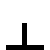7.8.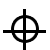9.10.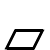11.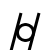12.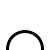13.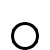14.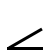15.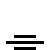16.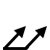17.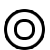18.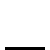19.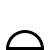20. What is a "Material Condition Modifier?"

21. A positional tolerance defines:

22. Which is the correct definition of regardless of feature size?

23. What is "Rule 1" (i.e. the "Envelope Rule" or the "Perfect form at MMC Rule"), and what effect does it have, if any, on flatness? ...straightness? ...perpendicularity? ...position?

24. What is the difference between a Datum and a Datum Feature?

25. What is a Datum Reference Frame?

26. What is MMC Virtual Condition?

### Refer to Figure 1 for the following questions: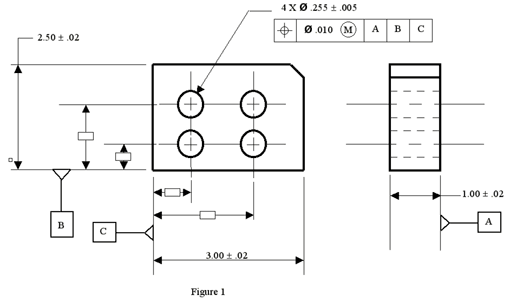27. What is the MMC size of one of the holes?

28. What is the MMC Virtual Condition size of one of the holes?

29. What is the LMC size of one of the holes?

30. How much total positional tolerance would be available for one hole if that hole was produced at a size of Ø.255?

### Refer to Figure 2 for the following questions: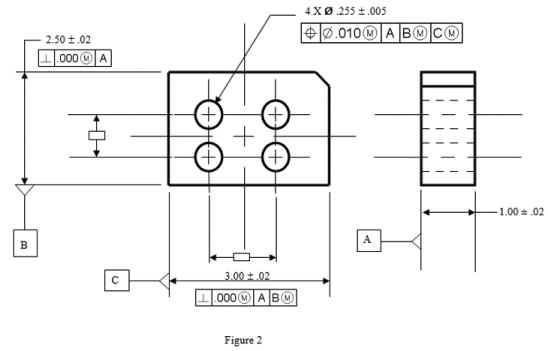31. Datum feature "B" was manufactured at a related actual mating size (RAME) of 2.49. Datum feature "C" was manufactured at an RAME of 3.01. Now how much total positional tolerance does that one hole (relative to the other holes in the pattern) have? (The considered hole is still Ø.255.)

32. With the same actual datum feature sizes and considered hole size defined in the previous question, how much extra tolerance does the 4-hole pattern have relative to the "center of the part?" (Datum features "B" and "C" define the "Center" of the part.)

### Complete the next 3 questions using the figure shown below:(all answers should be entered as “.XXX”.)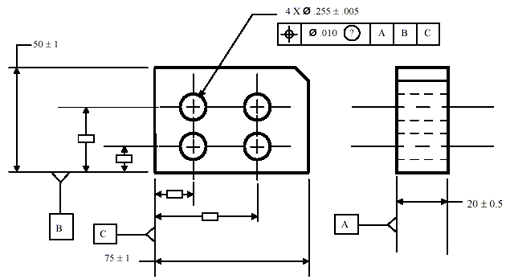#### Actual Manufactured Hole Size (measured) : Ø.250

33. Total Position Tolerance: When "?" = MØ____

34. Total Position Tolerance: When "?" = LØ____

35. Total Position Tolerance: When "?" is blank (no symbol) Ø____

### Complete the next 3 questions using the figure shown below:(all answers should be entered as “.XXX”.)#### Actual Manufactured Hole Size (measured) : Ø.251

36. Total Position Tolerance: When "?" = MØ____

37. Total Position Tolerance: When "?" = L – Ø____

38. Total Position Tolerance: When "?" is blank (no symbol)Ø____

### Complete the next 3 questions using the figure shown below:(all answers should be entered as “.XXX”.)#### Actual Manufactured Hole Size (measured) : Ø.252

39. Total Position Tolerance: When "?" = MØ____

40. Total Position Tolerance: When "?" = LØ____

41. Total Position Tolerance: When "?" is blank (no symbol) Ø____

### Complete the next 3 questions using the figure shown below:(all answers should be entered as “.XXX”.)#### Actual Manufactured Hole Size (measured) : Ø.253

42. Total Position Tolerance: When "?" = MØ____

43. Total Position Tolerance: When "?" = LØ____

44. Total Position Tolerance: When "?" is blank (no symbol)Ø____

### Complete the next 3 questions using the figure shown below:(all answers should be entered as “.XXX”.)#### Actual Manufactured Hole Size (measured) : Ø.254

45. Total Position Tolerance: When "?" = MØ____

46. Total Position Tolerance: When "?" = LØ____

47. Total Position Tolerance: When "?" is blank (no symbol)Ø____

### Complete the next 3 questions using the figure shown below:(all answers should be entered as “.XXX”.)#### Actual Manufactured Hole Size (measured) : Ø.255

48. Total Position Tolerance: When "?" = MØ____

49. Total Position Tolerance: When "?" = LØ____

50. Total Position Tolerance: When "?" is blank (no symbol)Ø____

### Complete the next 3 questions using the figure shown below:(all answers should be entered as “.XXX”.)#### Actual Manufactured Hole Size (measured) : Ø.258

51. Total Position Tolerance: When "?" = MØ____

52. Total Position Tolerance: When "?" = LØ____

53. Total Position Tolerance: When "?" is blank (no symbol)Ø____

### Complete the next 3 questions using the figure shown below:(all answers should be entered as “.XXX”.)#### Actual Manufactured Hole Size (measured) : Ø.260

54. Total Position Tolerance: When "?" = MØ____

55. Total Position Tolerance: When "?" = LØ____

56. Total Position Tolerance: When "?" is blank (no symbol)Ø____

57. The use of zero positional tolerance at MMC:

58. The use of the term, “BOUNDARY” under a position feature control frame:

59. A projected tolerance zone:

### Refer to Figure 4 for the following questions: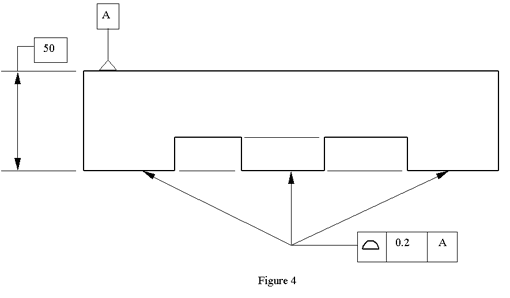60. Using Figure 4, how flat must the considered surfaces be (worst case) to meet the print requirements?

61. In Figure 4, if the actual of the overall height (when measuring the part against the 50mm specification) was 50.15, would that part be within the print specifications?

*Please enter the characters from above in the box below

*Full Name
Company Name